# SSC CGL 2018 Practice Test Papers | Quantitative Aptitude (Day-51)

Dear Aspirants, Here we have given the Important SSC CGL Exam 2018 Practice Test Papers. Candidates those who are preparing for SSC CGL 2018 can practice these questions to get more confidence to Crack SSC CGL 2018 Examination.

[WpProQuiz 3567]

Click “Start Quiz” to attend these Questions and view Explanation

1) A and B together can do a piece of work in 15 days. A alone started the work and worked 14 days for days and B finishes the remaining work in 16 days. How long B will take to complete the entire work?

a) 30

b) 25

c) 15

d) 12

2) Amrish is 100% more efficient than Baghvan and together they finish a piece of work in 180 days. In how many days will Sekar alone finish the work if efficiency of Sekar and Baghvan is in the ratio 3:1?

a) 60

b) 180

c) 90

d) 270

3) A glass kettle is marked up by 75% and 25% discount is offered for the first 50 customers. The cost price is Rs 16. What is the difference amount gained by 30th customer compared to 52nd customer?

a) 7

b) 10

c) 8

d) 5

4) What is the area (in sq cm) of a circle whose radius is equal to the breadth of the rectangle if diagonal of rectangle is 25 cm and length is 24 cm?

a) 414 sq.cm

b) 324 sq.cm

c) 120 sq.cm

d) 154 sq.cm

5)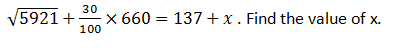a) 250

b) 350

c) 300

d) 400

6) A student scored 120 marks in the 5th internal test. Average marks of the student increases by 5 for every test. What will be his average after the 5th test?

a) 94

b) 95

c) 100

d) 80

7)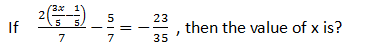a) 1/3

b) 2/3

c) 4/5

d) ½

8) What is the sum of the first 11 terms of an arithmetic progression if the second term is 15 and the 7th term is 5?

a) 66

b) 77

c) 33

d) 22

9)  −ax + 21y = 21 has slope of 4/7. What is the value of a?

a) 16

b) 12

c) 15

d) 17

10) What is the value of sec 45° – (1/√3) tan 60°?

a) √2+1

b) -√2+1

c) √2-1

d) None of the above

A+B=1/15

A’s 14 day work+ B’s 16 day work = 1

(A+B)’s 14 day work+ B’s 2 day work = 1

14/15+ B’s 2 day work = 1

B’s 2 day work = 1 – 14/15

B’s 2 day work = 1/15

B’s 1 day work = 1/ (15×2) = 1/30

B alone can do the work in 30 days

Efficiency of Amrish: Efficiency of Baghvan= 2:1(Since Amrish is 100 % more efficient than Baghvan)

Amrish and Baghvan together take 30 days to complete the work.

3 —- 180

1 —- ?

?= (3×180)/1=540days

Baghvan take 540 days to complete the work.

Efficiency ratio of Sekar and Baghvan= 3:1

1 —- 540

3 —- ?

?= 1×540/3 = 180 days

Cost Price = 16

Marked Price= 16x 175/100 = 28

Selling Price = Marked Price for the 52nd customer (∴ 25% discount is only for first 50 customers)

So Selling Price of 52nd customer = 28

Selling Price of 30th customer = 28×75/100 = 21

Difference of amount  = 28 – 21 = 7

Area of Rectangle = lb

Area of Circle = πr2

r = b

Diagonal = 25 cm2

Length = 24 cm2

Breath = √(252 – 242) =√ (625 – 576) Using Pythagoras theorem

Breath = √49 = 7 cm2

Area of circle= πr2

= 22/7×7×7

= 154 sq.cm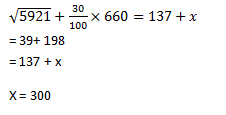So let the average of that Student for 4 tests be x

Average= (Total marks scored) / (Total tests)

Total marks scored in 4 testes = 4x

Now, he scores 120 in the 5th test

Total marks scored = 120 + 4x

After the 5th test his average increases by 5

Average = x + 5

Now equating the terms,

(120+4x)/5 =  x + 5

120 + 4x = 5x + 25

X = 95

So,

Average for 4 tests was 95

After 5th test average

= 95 + 5 = 100

(or)

5x – 4(x – 5) = 120

5x – 4x + 20 = 120

X = 100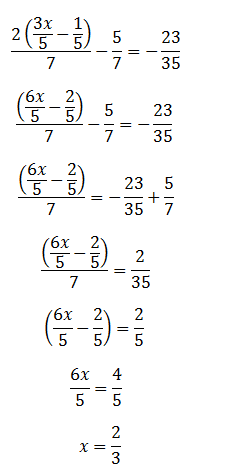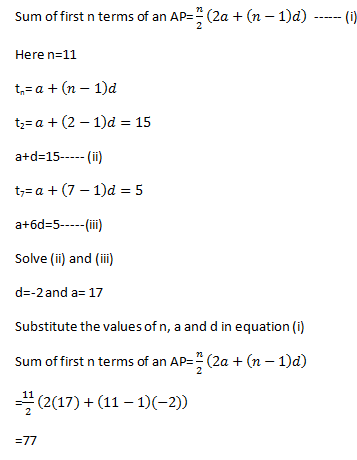– ax + 21y = 21

21y= ax+21

y= a/21 x+ 1—-(i)

Slope=m

m=4/7

Compare the equation (i) with y=mx+c

a/21=4/7

a=12